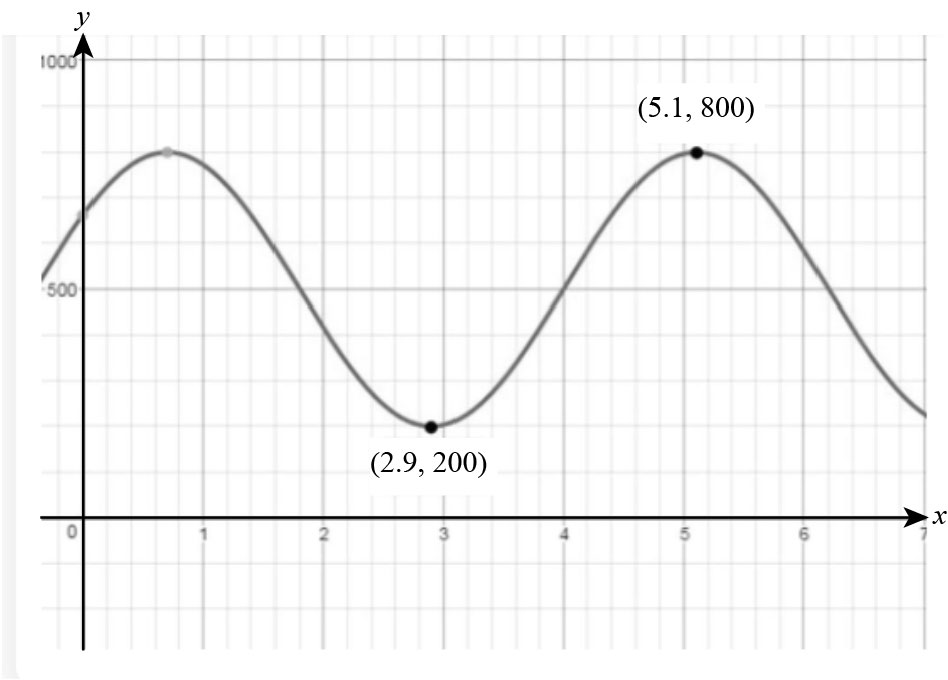×
Get Full Access to Precalculus With Trigonometry: Concepts And Applications - 1 Edition - Chapter 3-7 - Problem 2
Get Full Access to Precalculus With Trigonometry: Concepts And Applications - 1 Edition - Chapter 3-7 - Problem 2

×

# Fox Population Problem: Naturalists find that populations of some kinds of predatoryISBN: 9781559533911 468

## Solution for problem 2 Chapter 3-7

Precalculus with Trigonometry: Concepts and Applications | 1st Edition

• Textbook Solutions
• 2901 Step-by-step solutions solved by professors and subject experts
• Get 24/7 help from StudySoup virtual teaching assistantsPrecalculus with Trigonometry: Concepts and Applications | 1st Edition

4 5 1 307 Reviews
10
4
Problem 2

Fox Population Problem: Naturalists find that populations of some kinds of predatory animals vary periodically with time. Assume that the population of foxes in a certain forest varies sinusoidally with time. Records started being kept at time t = 0 years. A minimum number of 200 foxes appeared when t = 2.9 years. The next maximum, 800 foxes, occurred at t = 5.1 years. a. Sketch the graph of this sinusoid. b. Find a particular equation expressing the number of foxes as a function of time. c. Predict the fox population when t = 7, 8, 9, and 10 years. d. Foxes are declared an endangered species when their population drops below 30 Between what two nonnegative values of t were the foxes first endangered? e. Show on your graph in part a that your answer to part d is correct.

Step-by-Step Solution:

Step 1 of 4

a) Use the data given in the question to sketch the sinusoid as shown below:As seen, the population is minimum atyears and is maximum atyears.

Step 2 of 4

Step 3 of 4

##### ISBN: 9781559533911

Unlock Textbook Solution